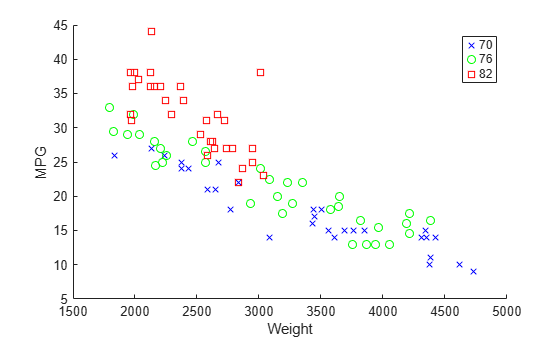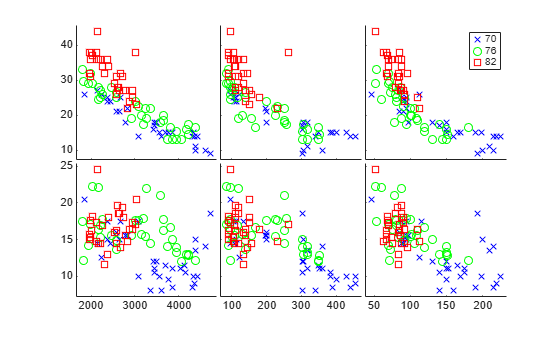# Create Scatter Plots Using Grouped Data

This example shows how to create scatter plots using grouped sample data.

A scatter plot is a simple plot of one variable against another. The MATLAB® functions `plot` and `scatter` produce scatter plots. The MATLAB function `plotmatrix` can produce a matrix of such plots showing the relationship between several pairs of variables.

Statistics and Machine Learning Toolbox™ functions `gscatter` and `gplotmatrix` produce grouped versions of these plots. These functions are useful for determining whether the values of two variables or the relationship between those variables is the same in each group. These functions use different plotting symbols to indicate group membership. You can use `gname` to label points on the plots with a text label or an observation number.

Suppose you want to examine the weight and mileage of cars from three different model years.

```load carsmall gscatter(Weight,MPG,Model_Year,'','xos')```This shows that not only is there a strong relationship between the weight of a car and its mileage, but also that newer cars tend to be lighter and have better gas mileage than older cars.

The default arguments for `gscatter` produce a scatter plot with the different groups shown with the same symbol but different colors. The last two arguments above request that all groups be shown in default colors and with different symbols.

The `carsmall` data set contains other variables that describe different aspects of cars. You can examine several of them in a single display by creating a grouped plot matrix.

```xvars = [Weight Displacement Horsepower]; yvars = [MPG Acceleration]; gplotmatrix(xvars,yvars,Model_Year,'','xos')```The upper right subplot displays `MPG` against `Horsepower`, and shows that over the years the horsepower of the cars has decreased but the gas mileage has improved.

The `gplotmatrix` function can also graph all pairs from a single list of variables, along with histograms for each variable. See MANOVA.